# 2020.3 月赛题解

oxx1108 edited 3 年前

#### 致歉

Xiejiadong ：由于出题人和验题人的疏忽。导致 $A$ 的 std 和数据是假的。

#### 花絮# Tag Idea Developer Tester
A - - - -
D 图论 树形 dp oxx1108 cubercsl Kilo_5723

### Problem B 迷宫

###### 解法 $2$

Weaver_zhu ：我想出了解法 $2$ 觉得挺真，就写了，然后就过了。

Xiejiadong ：想不出来为什么是对的，但是也过了压力测试，就是无法证明正确性，不知道大家有没有证明方法或者能 hack 掉它的数据。

### Problem D 排序树

###### 解法 $2$

• 原来想的是没有树这一限制，后来发现构造那一步会出环。比如这个数据:
4 4
1 3
1 4
2 3
2 4


• 对于按拓扑排序的层级顺序排序，然后添加相邻的边的方法，给一个反例：
4 3
1 3
2 4
3 4


### Problem E 钢琴演奏家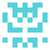PyPy3代码

p = 1000000007

def fastExpMod(b, e):
result = 1
while e != 0:
if (e&1) == 1:
result = (result * b) % p
e >>= 1
b = (b*b) % p
return result

for _ in range(int(input())):
n, m = map(int, input().split())
com = *(n+1)
com[m] = 1
for i in range(m+1, n+1):
com[i] = ((i*com[i-1]%p)*fastExpMod(i-m, p-2))%p
L = [int(i) for i in input().split()]
L.sort()
L = +L
res = 0
for i in range(n, m-1, -1):
res = (res+(((com[i]-com[i-1])*L[i])%p))%p
print(res)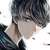#include <iostream>
#include <algorithm>

using namespace std;

typedef long long LL;

const int N = 1e6 + 7, mod = 1e9 + 7;

int a[N];
LL fact[N], infact[N]; // 阶乘数组最好用 long long 来存

// 快速幂
int qmi(int a, int k, int p) {
int res = 1;
while (k) {
if (k & 1) res = (LL)res * a % p;
a = (LL)a * a % p;
k >>= 1;
}
return res;
}

// 预处理阶乘
void init() {
fact = infact = 1;
for (int i = 1; i <= N; i++) {
fact[i] = fact[i - 1] * i % mod;
infact[i] = infact[i - 1] * qmi(i, mod - 2, mod) % mod;
}
}

int main() {
int T;
cin >> T;

init();

while (T --) {
int n, m;
cin >> n >> m;

for (int i = 1; i <= n; i++) {
cin >> a[i];
}

sort(a + 1, a + n + 1);

// 计算组合公式
int res = 0;
for (int i = m; i <= n; i ++) {
int x = i - 1, y = m - 1;
res = (res % mod + a[i] * 1ll * fact[x] % mod * infact[x - y] % mod * infact[y] % mod) % mod;
}
cout << res << endl;
}

return 0;
}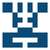### include

using namespace std;
typedef long long LL;
const LL N = 1000000007;
const int maxn = 1000000 + 10;
LL a[maxn];
LL fac[maxn];//乘阶表
LL FAC_K;
LL power(LL a, LL b) {//快速幂
a %= N;
LL ans = 1;
while (b) {
if (b & 1)ans = (ans * a) % N;
b >>= 1;
a = (a * a) % N;
}
return ans;
}
LL inv(LL a) {//返回逆元（费马小定理）
return power(a, N - 2L) % N;
}
void solve(int n) {//计算乘阶表
fac = 1;
for (int i = 1; i <= n; i++) {
fac[i] = (fac[i - 1] * i) % N;
}
}
LL comb(int n, int k) {//返回组合数（组合数公式）
if (k > n)return 0;
return ((fac[n] * FAC_K)% N * (inv(fac[n - k]) % N)) % N;
}

int main() {
int t;
scanf(“%d”, &t);
for (int i = 0; i < t; i++) {
int n, m;
scanf(“%d %d”, &n, &m);
for (int j = 0; j < n; j++) {
scanf(“%lld”, &a[j]);

    }
sort(a, a + n);
solve(n);
int require = m - 1;
FAC_K = inv(fac[require]);
long sum = 0;
for (int p = require; p < n; p++) {
sum = (sum % N + ((a[p] * comb(p, require)) % N)) % N;
}
sum %= N;
cout << sum << endl;
}
return 0;


}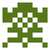E的考虑重复数据到底是什么意思啊 如果我脑子没抽的话题解里写的那句话应该前后矛盾才对……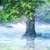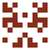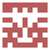1、测试集中是否一定无环呢？
2、假若某点在输入中从未出现，那么其入度为0，若将其作为拓扑排序的第一层是否可以内。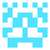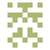E 题有 1000 组数据，但我代码每次处理阶乘要nlogn，这样复杂度会到1e9吧，可是为什么还能 AC